## Boltzmann’s constant is 1.38066×10⁻²³ J/K, and the universal gas constant is 8.31451 J/K · mol. If 3 mol of a gas is confined to a 6.1 L ves

Question

Boltzmann’s constant is 1.38066×10⁻²³ J/K, and the universal gas constant is 8.31451 J/K · mol. If 3 mol of a gas is confined to a 6.1 L vessel at a pressure of 7 atm, what is the average kinetic energy of a gas molecule? Answer in units of J.

in progress 0
2 months 2021-07-28T14:45:38+00:00 1 Answers 0 views 0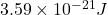Explanation:

We are given that

Boltzmann’s constant,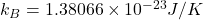Universal gas constant,R=8.31451 J/K

Number of moles,n=3

Volume ,V=6.1 L=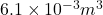Pressure,P=7 atm=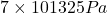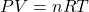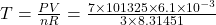T=173.45 K

Average kinetic energy=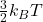Average kinetic energy=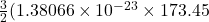Average kinetic energy=